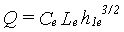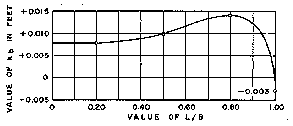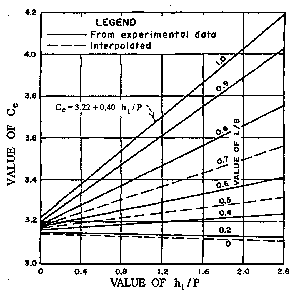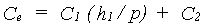CHAPTER 7 - WEIRS

6. Partially and Fully Contracted Rectangular Weirs

Kindsvater and Carter (1959) developed an improved method for calibration rating of rectangular thin-plate weirs. The method applies to both fully and partially contracted rectangular weirs. The method also rates the equivalent of a suppressed weir. The capability of rating partially contracted weirs provides design versatility, especially in selection of low crest heights to reduce head drop and side contraction needed to measure flow. Thus, these weirs can reduce head loss and conserve delivery head. These weirs have coefficients that vary with measuring head as well as geometry. The resulting calibrations are at least as accurate as the equations and tables for "standard" fully contracted weirs. Weir use and dimension limits are defined by the curves for determining the calibration ratings.

The basic equation for the Kindsvater-Carter method is:(7-1)

where:

Q = discharge, cubic feet per second (ft3/s)
e = a subscript denoting "effective"
Ce = effective coefficient of discharge, ft1/2/s
Le = L + kb
h1e = h1 + kh

In these relationships:

kb = a correction factor to obtain effective weir length
L = measured length of weir crest
B = average width of approach channel, ft
h1 = head measured above the weir crest, ft
kh = a correction factor with a value of 0.003 ft

The factor kb changes with different ratios of crest length, L, to average width of approach channel, B. Values of kb for ratios of L/B from 0 to 1 are given on figure 7-4. The factor kh is a constant value equal to 0.003 ft.Figure 7-4 -- Value of width-adjustment factor from Georgia Institute of Technology tests (courtesy of American Civil Society of Engineers).

The effective coefficient of discharge, Ce, includes effects of relative depth and relative width of the approach channel. Thus, Ce is a function of h1/p and L/B, and values of Ce may be obtained from the family of curves presented on figure 7-5. p is the vertical distance from the weir crest to the approach pool invert.Figure 7-5 -- Effective coefficient of discharge, Ce, as a function of L/B and h1/p, from Georgia Institute of Technology tests (courtesy of American Civil Society of Engineers)..

The straight lines on figure 7-5 have the equation form:(7-2)

where:

Ce = effective coefficient of discharge
C1 = equation coefficient
h1 = head on the weir (ft)
p = height of crest above approach invert (ft)
C2 = equation constant

For convenience, the coefficients and constants for straight lines of each L/B on figure 7-5 are given in the following tabulation for interpolation:

 Table 7-1. Coefficient and constants used in determining the effective coefficient of discharge for the Kindsvater-Carter method L/B C1 C2 0.2 0.4 0.5 0.6 0.7 0.8 0.9 1.0 -0.0087 0.0317 0.0612 0.0995 0.1602 0.2376 0.3447 0.4000 3.152 3.164 3.173 3.178 3.182 3.189 3.205 3.220

The straightforward, comprehensive, and accurate Kindsvater-Carter method of determining discharges for rectangular weirs is well suited for discharge rating use. It is particularly useful for installations where full crest contractions or full end contractions are difficult to achieve.

Traditional rectangular weirs that do not meet crest height limits or that are using the older methods of correcting for velocity of approach should be recalibrated using the Kindsvater-Carter method. Weirs that fall out of the limits of the Kindsvater-Carter rating curves need replacement or field calibration by thorough current metering.

Limits on usage and dimensions are:

• The calibration relationships were developed with rectangular approach flow and head measurement sections for these weirs. For applications with other flow section shapes, the average width of the flow section for each h1 is used as B to calculate discharges.
• The crest length, L, should be at least 6 in.
• The crest height, p, should be at least 4 in.
• Like all weirs used for head measurement, h1 should be at least 0.2 ft .
• Values of h1/p should be less than 2.4.
• All the requirements in section 5 apply.
• The downstream water surface elevation should be at least 2 in below the crest.
• All the approach flow conditions in chapter 2 apply .